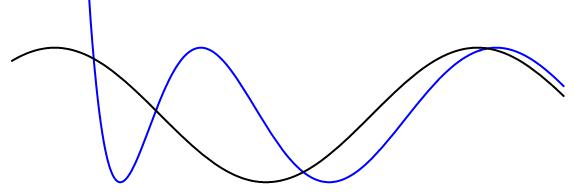# Cosine and Something Else

Geometry Level 5

Let $n$ be a positive nonzero integer and$k=4n,$
$a=x+\sqrt { { x }^{ 2 }-{ k }^{ 2 } },$
$b=x-\sqrt { { x }^{ 2 }-{ k }^{ 2 } },$
$f\left( x \right) =\dfrac { 1 }{ 2 } \dfrac { 1 }{ { k }^k } } \left( { a }^{ \displaystyle k }+{ b }^k } \right)$

For what integer $n<1000$ does $f\left( x \right)$ intersect $\cos\left( x \right)$ the most times between $-4\pi ?

You may want to use a graphing calculator.

×

Problem Loading...

Note Loading...

Set Loading...